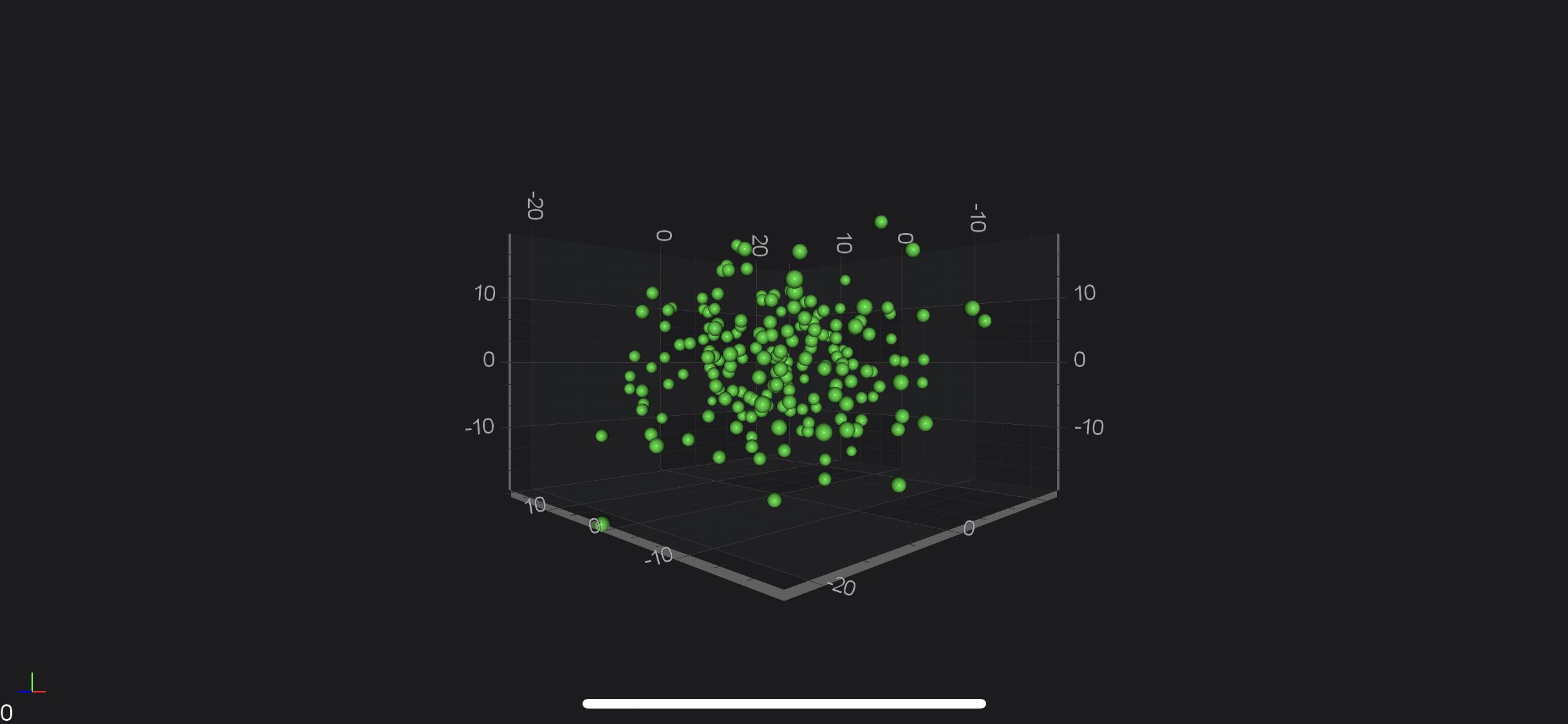iOS & macOS Charting Documentation - SciChart iOS & macOS Charts SDK v4.x

# SciChart iOS 3D Tutorial - Create a simple Scatter Chart 3D

In this SciChart iOS 3D tutorial, you’ll learn to:

NOTE: This tutorial assumes that you’ve already know how to Link SciChart iOS and Add SCIChartSurface3D instance into your `ViewController`. If you need more information - please read the following articles:

## Getting Started

This tutorial is suitable for Objective-C, Swift and C# with Xamarin.iOS.

NOTE: Source code for this tutorial can be found at our Github Repository:

## Adding 3D Axes to the SCIChartSurface3D

Once you have added a `SCIChartSurface3D` into your ViewController, you will not see anything drawn because you need to add axes. This is important: that three axes X, Y and Z has to be added to your surface.This is a bare minimum to see drawn grid on your device.

self.surface.xAxis = [SCINumericAxis3D new]; self.surface.yAxis = [SCINumericAxis3D new]; self.surface.zAxis = [SCINumericAxis3D new];
self.surface.xAxis = SCINumericAxis3D() self.surface.yAxis = SCINumericAxis3D() self.surface.zAxis = SCINumericAxis3D()
Surface.XAxis = new SCINumericAxis3D(); Surface.YAxis = new SCINumericAxis3D(); Surface.ZAxis = new SCINumericAxis3D();Now, we would like to see something more than just empty grid, e.g. Scatter 3D Chart. To draw some data in chart we need to create 3D DataSeries and RenderableSeries. The DataSeries is a class which is responsible for storing data which should be displayed and RenderableSeries is a class that determines how data should be visualized by chart.

First, let’s declare the 3D DataSeries and generate some data for it:

SCIXyzDataSeries3D *ds = [[SCIXyzDataSeries3D alloc] initWithXType:SCIDataType_Double yType:SCIDataType_Double zType:SCIDataType_Double]; for (int i = 0; i < 200; ++i) { double x = [self getGaussianRandomNumber:5 stdDev:1.5]; double y = [self getGaussianRandomNumber:5 stdDev:1.5]; double z = [self getGaussianRandomNumber:5 stdDev:1.5]; [ds appendX:@(x) y:@(y) z:@(z)]; }
let dataSeries = SCIXyzDataSeries3D(xType: .double, yType: .double, zType: .double) for _ in 0 ..< 200 { let x = getGaussianRandomNumber(mean: 5, stdDev: 1.5) let y = getGaussianRandomNumber(mean: 5, stdDev: 1.5) let z = getGaussianRandomNumber(mean: 5, stdDev: 1.5) dataSeries.append(x: x, y: y, z: z) }
var dataSeries3D = new XyzDataSeries3D<double, double, double>(); for (int i = 0; i < 200; i++) { double x = GetGaussianRandomNumber(5, 1.5); double y = GetGaussianRandomNumber(5, 1.5); double z = GetGaussianRandomNumber(5, 1.5); dataSeries3D.Append(x, y, z); }

The next step is to create 3D RenderableSeries. In our case we’re going to display data as a point cloud using Scatter Series 3D, which is represented by the `SCIScatterRenderableSeries3D`:

SCISpherePointMarker3D *pointMarker = [SCISpherePointMarker3D new]; pointMarker.fillColor = 0xFF32CD32; pointMarker.size = 10.0; SCIScatterRenderableSeries3D *rSeries = [SCIScatterRenderableSeries3D new]; rSeries.dataSeries = dataSeries; rSeries.pointMarker = pointMarker;
let pointMarker = SCISpherePointMarker3D() pointMarker.fillColor = 0xFF32CD32; pointMarker.size = 10.0 let rSeries = SCIScatterRenderableSeries3D() rSeries.dataSeries = dataSeries rSeries.pointMarker = pointMarker
var rSeries = new SCIScatterRenderableSeries3D { DataSeries = dataSeries, PointMarker = new SCISpherePointMarker3D { FillColor = 0xFF32CD32, Size = 10.0f }, };

Finally, we need to add newly created series into the `ISCIChartSurface3D.renderableSeries` collection, like so:

[SCIUpdateSuspender usingWithSuspendable:self.surface withBlock:^{ self.surface.xAxis = [SCINumericAxis3D new]; self.surface.yAxis = [SCINumericAxis3D new]; self.surface.zAxis = [SCINumericAxis3D new]; [self.surface.renderableSeries add:rSeries]; }];
SCIUpdateSuspender.usingWith(self.surface) { self.surface.xAxis = SCINumericAxis3D() self.surface.yAxis = SCINumericAxis3D() self.surface.zAxis = SCINumericAxis3D() self.surface.renderableSeries.add(rSeries) }
using (Surface.SuspendUpdates()) { Surface.XAxis = new SCINumericAxis3D(); Surface.YAxis = new SCINumericAxis3D(); Surface.ZAxis = new SCINumericAxis3D(); Surface.RenderableSeries.Add(rSeries); }

Which will render the following Scatter Chart 3D:NOTE: Please note that we’ve added axes and renderableSeries to `SCIChartSurface3D` inside `+[SCIUpdateSuspender usingWithSuspendable:withBlock:]` block. This allows to suspend surface instance and refresh it only one time after you finished all needed operations. That’s highly recommended technique, if you want to omit performance decrease due to triggering refreshes on every operation which could be performed in one batch.